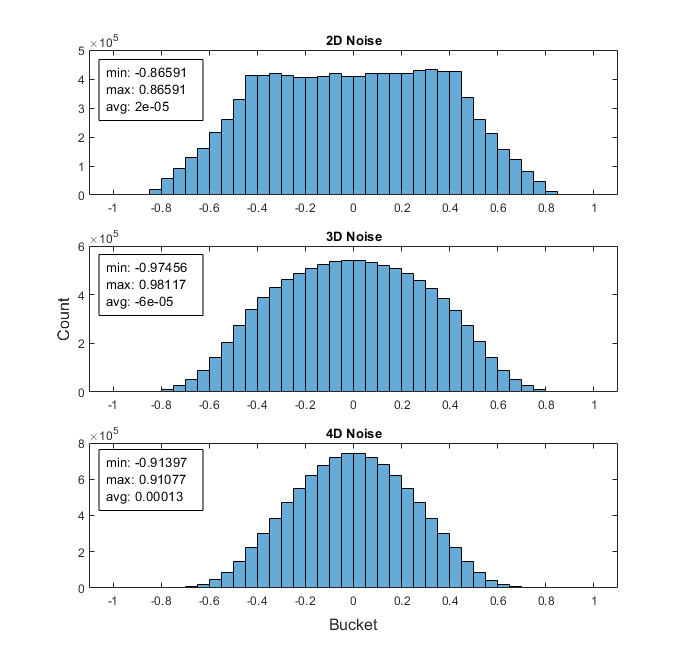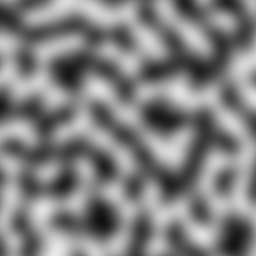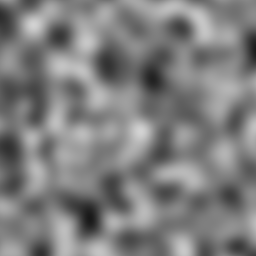OpenSimplex is a noise generation function like Perlin or Simplex noise, but better.

# OpenSimplex Noise

OpenSimplex is a noise generation function like Perlin or Simplex noise, but better.

OpenSimplex noise is an n-dimensional gradient noise function that was
developed in order to overcome the patent-related issues surrounding
Simplex noise, while continuing to also avoid the visually-significant
directional artifacts characteristic of Perlin noise.
- Kurt Spencer


This is merely a python port of Kurt Spencer's original code (released to the public domain) and neatly wrapped up in a package.

## Status

The master branch contains the latest code (possibly unstable), with automatic tests running for Python 3.8, 3.9, 3.10 on Linux, MacOS and Windows. FreeBSD is also supported, though it's only locally tested as Github Actions don't offer FreeBSD support.

Please refer to the version tags for the latest stable version.

• Adds a hard dependency on 'Numpy', for array optimizations aimed at heavier workloads.
• Adds optional dependency on 'Numba', for further speed optimizations using caching (currently untested due to issues with llvmlite).
• General refactor and cleanup of the library, tests and docs.
• Breaking changes: API functions uses new names.

## Contributions

Bug reports, bug fixes and other issues with existing features of the library are welcomed and will be handled during the maintainer's free time. New stand-alone examples are also accepted.

However, pull requests with new features for the core internals will not be accepted as it eats up too much weekend time, which I would rather spend on library stability instead.

## Usage

Installation

pip install opensimplex


Basic usage

>>> import opensimplex
>>> opensimplex.seed(1234)
>>> n = opensimplex.noise2(x=10, y=10)
>>> print(n)
0.580279369186297


Running tests and benchmarks

Setup a development environment:

make dev
source devenv/bin/activate
make deps


And then run the tests:

make test


Or the benchmarks:

make benchmark


For more advanced examples, see the files in the tests and examples directories.

## API

opensimplex.seed(seed)

Seeds the underlying permutation array (which produces different outputs),
using a 64-bit integer number.
If no value is provided, a static default will be used instead.

seed(13)


random_seed()

Works just like seed(), except it uses the system time (in ns) as a seed value.
Not guaranteed to be random so use at your own risk.

random_seed()


get_seed()

Return the value used to seed the initial state.
:return: seed as integer

>>> get_seed()
3


opensimplex.noise2(x, y)

Generate 2D OpenSimplex noise from X,Y coordinates.
:param x: x coordinate as float
:param y: y coordinate as float
:return:  generated 2D noise as float, between -1.0 and 1.0

>>> noise2(0.5, 0.5)
-0.43906247097569345


opensimplex.noise2array(x, y)

Generates 2D OpenSimplex noise using Numpy arrays for increased performance.
:param x: numpy array of x-coords
:param y: numpy array of y-coords
:return:  2D numpy array of shape (y.size, x.size) with the generated noise
for the supplied coordinates

>>> rng = numpy.random.default_rng(seed=0)
>>> ix, iy = rng.random(2), rng.random(2)
>>> noise2array(ix, iy)
array([[ 0.00449931, -0.01807883],
[-0.00203524, -0.02358477]])


opensimplex.noise3(x, y, z)

Generate 3D OpenSimplex noise from X,Y,Z coordinates.
:param x: x coordinate as float
:param y: y coordinate as float
:param z: z coordinate as float
:return:  generated 3D noise as float, between -1.0 and 1.0

>>> noise3(0.5, 0.5, 0.5)
0.39504955501618155


opensimplex.noise3array(x, y, z)

Generates 3D OpenSimplex noise using Numpy arrays for increased performance.
:param x: numpy array of x-coords
:param y: numpy array of y-coords
:param z: numpy array of z-coords
:return:  3D numpy array of shape (z.size, y.size, x.size) with the generated
noise for the supplied coordinates

>>> rng = numpy.random.default_rng(seed=0)
>>> ix, iy, iz = rng.random(2), rng.random(2), rng.random(2)
>>> noise3array(ix, iy, iz)
array([[[0.54942818, 0.54382411],
[0.54285204, 0.53698967]],
[[0.48107672, 0.4881196 ],
[0.45971748, 0.46684901]]])


opensimplex.noise4(x, y, z, w)

Generate 4D OpenSimplex noise from X,Y,Z,W coordinates.
:param x: x coordinate as float
:param y: y coordinate as float
:param z: z coordinate as float
:param w: w coordinate as float
:return:  generated 4D noise as float, between -1.0 and 1.0

>>> noise4(0.5, 0.5, 0.5, 0.5)
0.04520359600370195


opensimplex.noise4array(x, y, z, w)

Generates 4D OpenSimplex noise using Numpy arrays for increased performance.
:param x: numpy array of x-coords
:param y: numpy array of y-coords
:param z: numpy array of z-coords
:param w: numpy array of w-coords
:return:  4D numpy array of shape (w.size, z.size, y.size, x.size) with the
generated noise for the supplied coordinates

>>> rng = numpy.random.default_rng(seed=0)
>>> ix, iy, iz, iw = rng.random(2), rng.random(2), rng.random(2), rng.random(2)
>>> noise4array(ix, iy, iz, iw)
array([[[[0.30334626, 0.29860705],
[0.28271858, 0.27805178]],
[[0.26601215, 0.25305428],
[0.23387872, 0.22151356]]],
[[[0.3392759 , 0.33585534],
[0.3343468 , 0.33118285]],
[[0.36930335, 0.36046537],
[0.36360679, 0.35500328]]]])


## FAQ

• What does the distribution of the noise values look like?• Is this relevantly different enough to avoid any real trouble with the original patent?

If you read the patent claims:

Claim #1 talks about the hardware-implementation-optimized gradient generator. Most software implementations of Simplex Noise don't use this anyway, and OpenSimplex Noise certainly doesn't.

Claim #2(&3&4) talk about using (x',y',z')=(x+s,y+s,z+s) where s=(x+y+z)/3 to transform the input (render space) coordinate onto a simplical grid, with the intention to make all of the "scissor-simplices" approximately regular. OpenSimplex Noise (in 3D) uses s=-(x+y+z)/6 to transform the input point to a point on the Simplectic honeycomb lattice so that the simplices bounding the (hyper)cubes at (0,0,..,0) and (1,1,...,1) work out to be regular. It then mathematically works out that s=(x+y+z)/3 is needed for the inverse transform, but that's performing a different (and opposite) function.

Claim #5(&6) are specific to the scissor-simplex lattice. Simplex Noise divides the (squashed) n-dimensional (hyper)cube into n! simplices based on ordered edge traversals, whereas OpenSimplex Noise divides the (stretched) n-dimensional (hyper)cube into n polytopes (simplices, rectified simplices, birectified simplices, etc.) based on the separation (hyper)planes at integer values of (x'+y'+z'+...).

Another interesting point is that, if you read all of the claims, none of them appear to apply to the 2D analogue of Simplex noise so long as it uses a gradient generator separate from the one described in claim #1. The skew function in Claim #2 only applies to 3D, and #5 explicitly refers to n>=3.

And none of the patent claims speak about using surflets / "spherically symmetric kernels" to generate the "images with texture that do not have visible grid artifacts," which is probably the biggest similarity between the two algorithms.

## Credits

• Kurt Spencer - Original work
• Owen Raccuglia - Test cases, Go Module
• /u/redblobgames - Fixed conversion for Java's long type, see Reddit

And all the other Github Contributors and Bug Hunters. Thanks!

While the original work was released to the public domain by Kurt, this package is using the MIT license.

## Example Output

More example code and trinkets can be found in the examples directory.

Example images visualising 2D, 3D and 4D noise on a 2D plane, using the default seed:

2D noise3D noise4D noise## Project details

This version0.4.50.4.40.4.30.4.20.40.30.20.1

Uploaded source
Uploaded py3